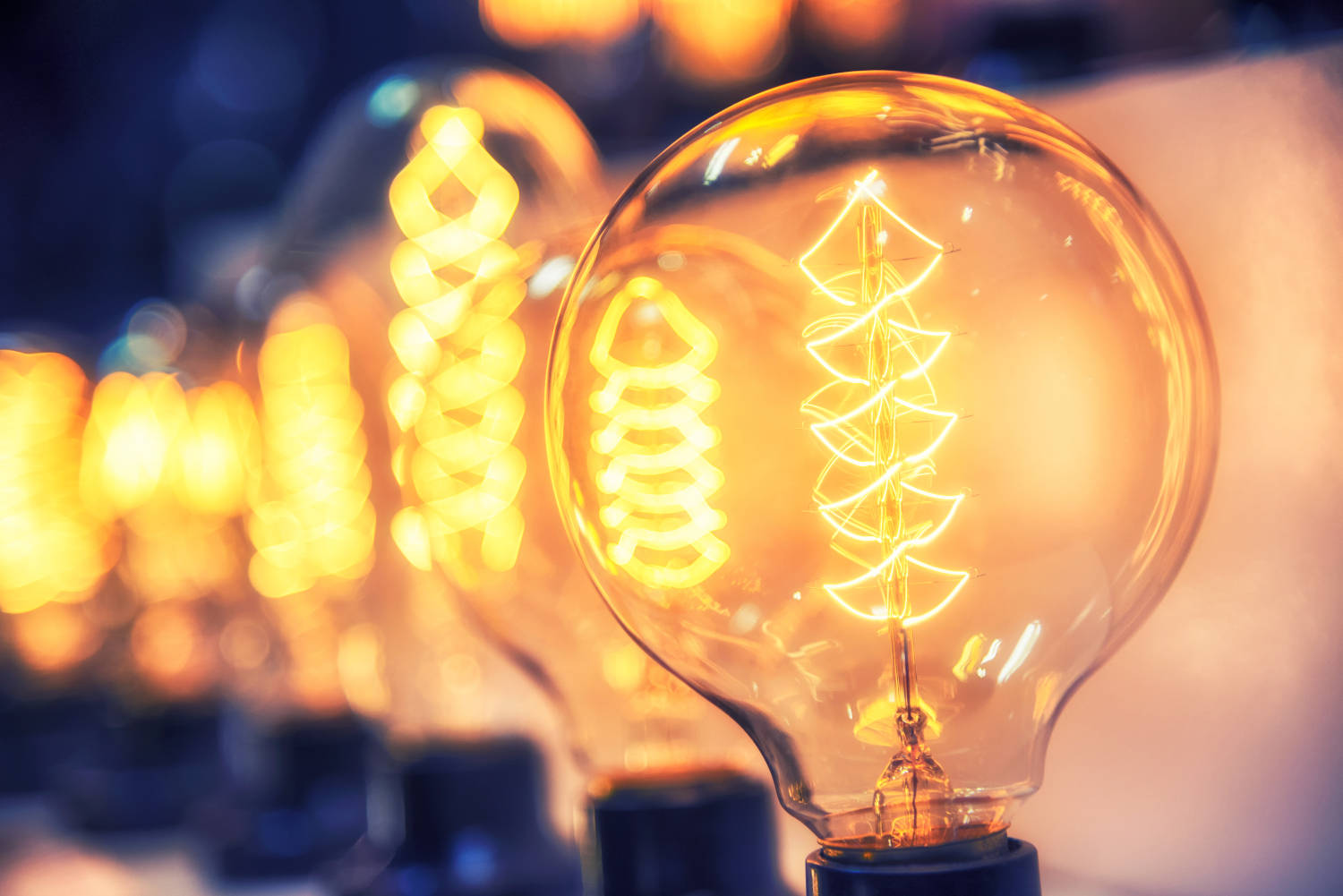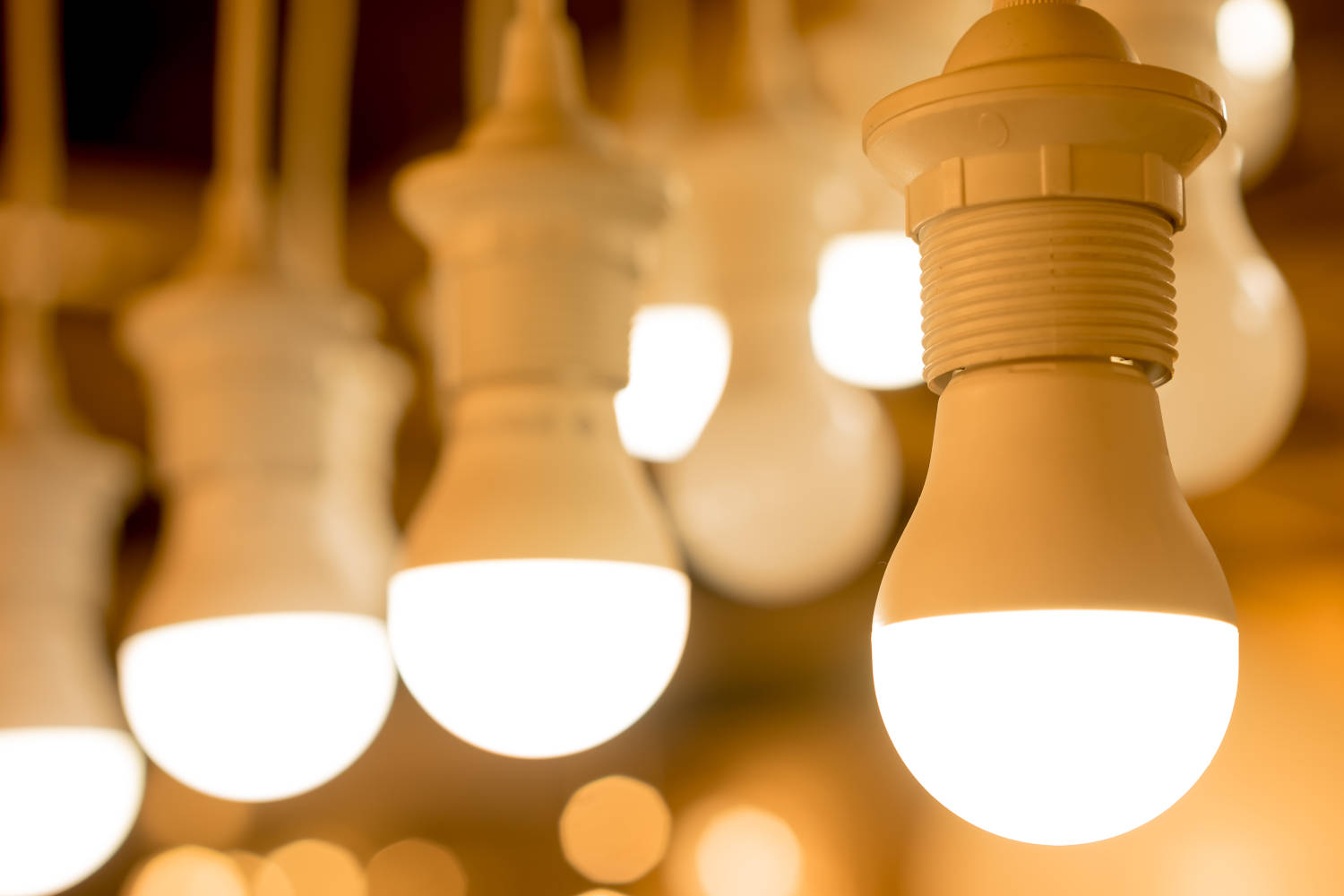# 2023 Lighting Energy Cost Calculator

Calculate the electricity costs of a light bulb or light fixture. Enter the number and wattage of the lamps and the average amount of time they are on. Find out how much electricity the light fixtures in your home are using and also estimate the monthly cost per light fixture.

watts
hours
\$
The average price of electricity is 14 cents per kWh

Estimate the cost savings and payback period for an upgrade to LED or CFL lamps (optional)

Replacement Lamp Details:
watts
\$

## Lighting Energy Cost Estimate:

### Electricity Costs per Month

 Before Replacing Lamps: \$2.02 After Replacing Lamps: \$0.29 Savings: \$1.73

### Payback Timeline

 Total Replacement Cost: \$5.00 Payoff Period: 3 months

### Electrical Usage per Month

 Before Replacing Lamps: 14.4 kWh After Replacing Lamps: 2.04 kWh Savings: 12.36 kWh
Learn how we calculated this below

## How to Estimate How Much a Light Bulb Costs

Find out exactly how much it costs to turn on the lights and see if it’s worth the upgrade to cost-saving LED or fluorescent lighting. The easiest way is to use the calculator above, it takes out all of the math and simplifies the process.

It’s also possible to find out how much lights cost to power using a few simple steps. Continue reading to learn a couple easy formulas to estimate lighting electricity costs.### Calculate the Total Wattage of a Light Fixture

The first step is to find the total wattage of the lights. Start by finding the wattage of each light bulb. This is often printed right on the light bulb or the original package. The average A19 incandescent light bulb uses 60 watts of electricity, which is a good starting point if the bulb is inaccessible.

To find the total wattage of a fixture, add the wattage of each light bulb together.

fixture watts = bulb watts × bulb quantity

For example, let’s find the wattage of a light fixture that uses three 60 W incandescent light bulbs.

fixture watts = 60 W × 3
fixture watts = 180 W

### Calculate the Energy Used for Lighting

The next step in estimating lighting costs is to find how much energy the lights consume. Find the energy used in kilowatt hours (kWh) by multiplying the total wattage for the fixture by the hours per day that the lights are on, then, divide this by 1,000.

Or, just use our watts to kWh calculator.

kWh = Power(W) × Time(hrs) ÷ 1,000

To determine the monthly cost, multiply the energy used per day by 30 to get the monthly kilowatt hours used.

monthly kWh = kWh per day × 30

For example, let’s find the kilowatt hours (kWh) used for a fixture with three 60 W light bulbs that is on for 8 hours per day.

Watts = 60 W × 3 = 180 W
kWh = 180 W × 8 hrs ÷ 1,000
kWh = 1.44 kWh

To find the monthly energy usage, multiply the result by 30.

monthly kWh = 1.44 kWh × 30
monthly kWh = 43.2 kWh

### Calculate the Total Electricity Cost for Lights

Calculate the total lighting cost by multiplying the kilowatt-hours used by the cost per kWh.

price = kWh × cost per kWh

Every electric company charges a different rate for electricity, and those rates may vary month to month. The national average rate for electricity is 13.87 cents per kilowatt-hour. Find the exact rate charged on the electric bill sent by the power company.

For example, continuing the previous example of the cost for a light fixture with three bulbs that is on for 8 hours per day, let’s find the monthly cost.

price = kWh × cost per kWh
price = 43.2 kWh × \$0.1387
kWh = \$5.99

That’s nearly \$6 per month for a single fixture with three light bulbs. Use our electricity cost calculator to determine the actual cost of electricity usage.

## How Much Money Can You Save Upgrading to LED Light Bulbs?

You’re probably surprised by how much it costs to power an incandescent light bulb. Multiply that by an entire home full of lights, and you may be shocked by how much that costs per month.

Enter LED and CFL light fixtures. These light bulbs are becoming very popular for their energy savings and longevity, but they do cost more than an incandescent light fixture. The energy cost calculator can estimate how long it will take to pay for an LED/CFL upgrade based on the amount of usage, electricity costs, and the number of lamps needed.In the past LED bulbs have had a poor reputation for bright or blue color temperatures and unnatural feel.

Modern-day LED bulbs are offered in a range of color temperatures from very cool in color to the warm glow of an incandescent that most are used to. Dimmable LED bulbs are also available.

An average LED light bulb that outputs the equivalent of the 60w incandescent fixture uses roughly 8-9 watts. Upgrading five light bulbs to LEDs using 9 watts of electricity would likely cost \$1.30 to power for the month vs. the \$8.70 of the incandescent lamps. That’s a significant cost savings.

LED lamps last significantly longer than incandescent lamps, meaning big savings by not needing to replace light bulbs every year or two. This is not as simple to estimate since light bulbs can last from 6 months to several years, but it’s obvious that over a lifetime an LED or CFL bulb pays for itself purely on replacement cost savings. The average LED bulb lasts 50,000-100,000 hours. A CFL light bulb lasts 25,000 hours on average.

## References

1. U.S. Energy Information Administration, Table 5.6.A. Average Price of Electricity to Ultimate Customers by End-Use Sector, July 2022, https://www.eia.gov/electricity/monthly/epm_table_grapher.php?t=epmt_5_6_a